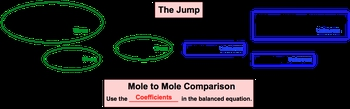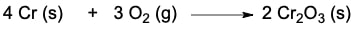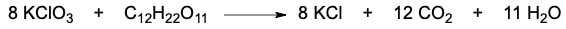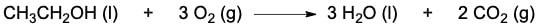## General Chemistry

Learn the toughest concepts covered in Chemistry with step-by-step video tutorials and practice problems by world-class tutors

3. Chemical Reactions

# Stoichiometry

Stoichiometry deals with the numerical relationship between compounds in a balanced chemical equation.

Stoichiometry
1
concept

## Stoichiometry1m
Play a video:
when it comes to chemical equations, it becomes important to first balance your chemical equation because this will later on lead us to our understanding of story geometry. Now, story geometry deals with the numerical relationship between compounds in a balanced chemical equation. It allows us to determine the amount of products from reactant and vice versa. For example, here we have our chemical equation. That's balance we have to Hydrogen gas is reacting with one oxygen gas to produce to water molecules as gas. They're giving us 12.3 g of H two, and they're asking us to determine the grams of H 20 H. 20 gas produced. This is what store geometry is. They're giving us a balanced chemical equation. They're giving us information on one of the compounds within this balanced equation and asking us to find another compound within that same balanced equation. Now that we know what's dark geometry is, how exactly do we do it? Well, if you click on the next video, we'll take a look at the procedures that you'll need to employ in order to do any type of stoke geometric calculation.
2
concept

## Stoichiometry3m
Play a video:
So as we said, we're given 12.3 g of H two. And as to find how many grams of H 20 would form the way we're able to do this is through stock geometry and to find how many grams would use these Tokyo metric chart. Now, the chart uses the given quantity of a compound to determine the unknown quantity of another compound. The way it works is we're starting here on the left side and on the left side. The information they give to us is called the Given Information. Now this information that's given to us can be presented as grams and if it's presented his grams, then we can just do a conversion to get two moles so we can go from grams of given two moles of given now. Besides giving it to us in grams, it could be given to us in ions, Adams formal units no or molecules of given. Again. We're heading towards moles of given, so our basic movement is going from the left side of the chart. Towards the right side of the chart toe are unknown information. Now that we have our moles of given, we'd have to basically take a jump, a leap of faith from an area where we know information because it's given to us tow a area where we know nothing at all. So it's unknown to us and this jumper leap of faith that we're going to take. We call it the jump. To be able to do this jump, you have to do a mole to mole comparison and in a multiple comparison, use the coefficients in the balanced equation. That's why it's incredibly important. You first have a balanced equation before you even attempt to do so. A geometry once we've gone from moles of given, two moles of unknown And then we can either stop there if they want us to find the answer in moles or continue onward to ions, Adams formula units or molecules of unknown or toe grams of unknown. In this case, from the example that we have up above of our equation, we would say that this is the grams given to us, so this would be our grams off given and we'd have to basically take the trip of grams of given two moles of Given. Two moles of our unknown are unknown is what we're looking for, which would be water, and then we'd have to stop here at grams of unknown. That's the path we have to take. We utilize this Tokyo metric chart to help us answer any type of stoking metric question. Now that we've seen this chart, move on to our example question and let's put it to work put into practice in terms of this story geometric chart.

Utilize the stoichiometric chart to do all your stoichiometric calculations.3
example

## Stoichiometry Example 15m
Play a video:
4
Problem

The oxidation of chromium solid is represented by the following equation:How many moles of chromium (III) oxide are produced when 34.69 g Cr reacts with excess oxygen gas?

5
Problem

The reaction of potassium chlorate and sucrose is given below:If 2.33 x 10-7 formula units of potassium chlorate are reacted, how many grams of carbon dioxide will be produced?

6
Problem

If the density of ethanol, CH3CH2OH, is 0.789 g/mL, how many milliliters of ethanol are needed to produce 4.8 g of H2O in the following reaction?